# Fast Sorting with Quicksort

by kirupa   |   filed under Algorithms and Data Structures

When it comes to sorting stuff, one of the most popular algorithms you have is quicksort. It is popular because it is fast - really fast when compared to other algorithms for similar types of workloads. Key to its speed is that quicksort is a divide-and-conquer algorithm. It is called that because of how it breaks up its work. Instead of eating a giant chunk of data in one bite and chewing it over a long period of time (kinda like an anaconda), quicksort breaks up its data into smaller pieces and chews on each smaller piece quickly.

In this ridiculously long article, we'll go a tad bit more precise and really understand how quicksort works. By the end of this, you'll be able to regale your friends and family (over dinner, preferably) with all the cool things quicksort does. You may even improve your code with this knowledge.

Onwards!

## A Look at How Quicksort Works

Like I mentioned earlier, quicksort works by dividing the input into several smaller pieces. On these smaller pieces, it does its magic by a combination of further dividing the input and sorting the leftovers. This is a pretty tough thing to explain in one attempt, so I will first provide a simple overview of how quicksort works, and then I will provide a more detailed look at how the major parts work by showing you some pseudocode and walking through a small example. If you are still awake after all that, we will take a look at a fully-working quicksort implementation that puts into code all of the text and diagrams that you are about to see.

### A Simple Look

Let's imagine that the following grid of squares represents the numbers we want to sort:Our goal is to sort these numbers, and we are going to be using quicksort to help us with the sorting. No surprises so far. When quicksort starts running at full speed, it broadly does three things:

1. Picks a point that other numbers will rally around.
2. Re-orders items based on how large they are relative to the pivot.
1. Items smaller than the pivot are moved to the left of the pivot
2. Items larger than the pivot are moved to the right of the pivot
3. Repeats Steps i and ii on the values partially sorted thanks to Step ii.

At first glance, how these three steps help you sort some data may seem bizarre, but let's walk through this example to see these steps at work.

Starting at the top, the way quicksort works is by first picking an item in your list of items called the pivot: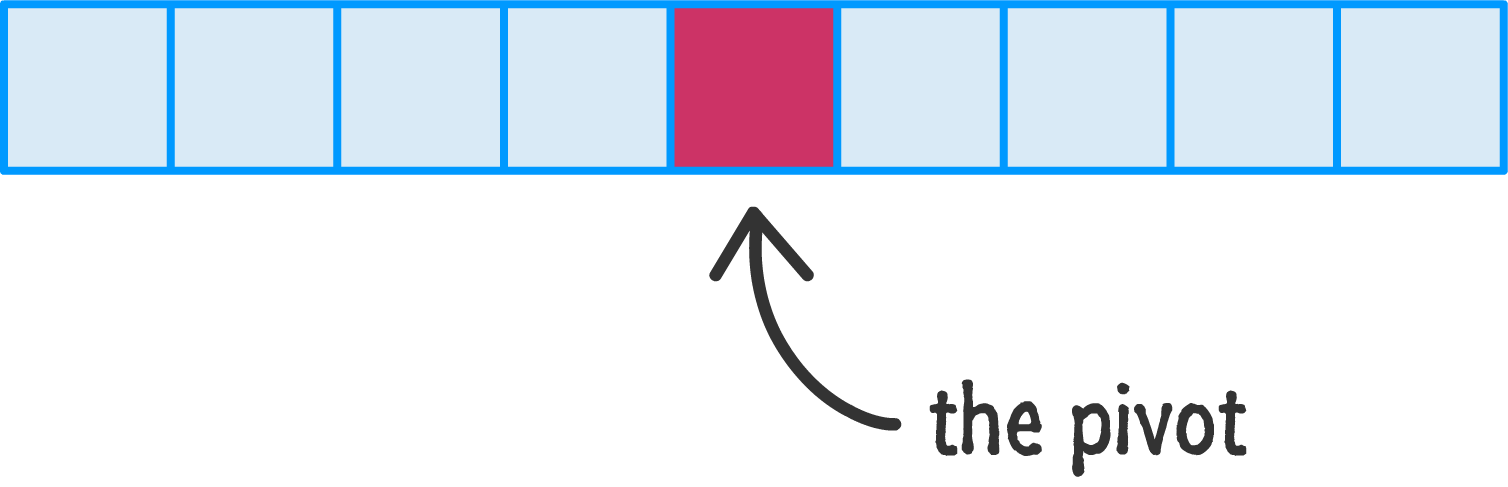You can pick your pivot from anywhere, but all the cool kids pick (for various good reasons) the pivot from the midpoint. Since we want to be cool as well, that's what we'll do. Anyway, quicksort uses the pivot value to order items in a very crude and basic way. From quicksort's point of view, all items to the left of the pivot value should be smaller, and all items to the right of the pivot value should be larger:Values are simply thrown to either side of the pivot depending on how large they are compared to the pivot. Here is a visualization of what this might look like if I gave our values a height:The thing to emphasize is that the values to the left and right of the pivot are not ordered. They are simply smaller or larger. The only guarantee you have is that the values to the left of the pivot are smaller than the pivot, and the other guarantee you have is that the values to the right of the pivot are larger. The other OTHER guarantee you have is that your pivot is at this point in the perfect arrangement. No matter what happens to the arrangement of the other values, they will never dislodge your current pivot value from its current position. Your pivot is in its final, sorted position.

After picking your first pivot and throwing larger and smaller values to either side of it, you now have two sections of data on either side of this pivot that are only partially sorted. What you do next is repeat all of this pivot picking and re-arranging on each of these two sections: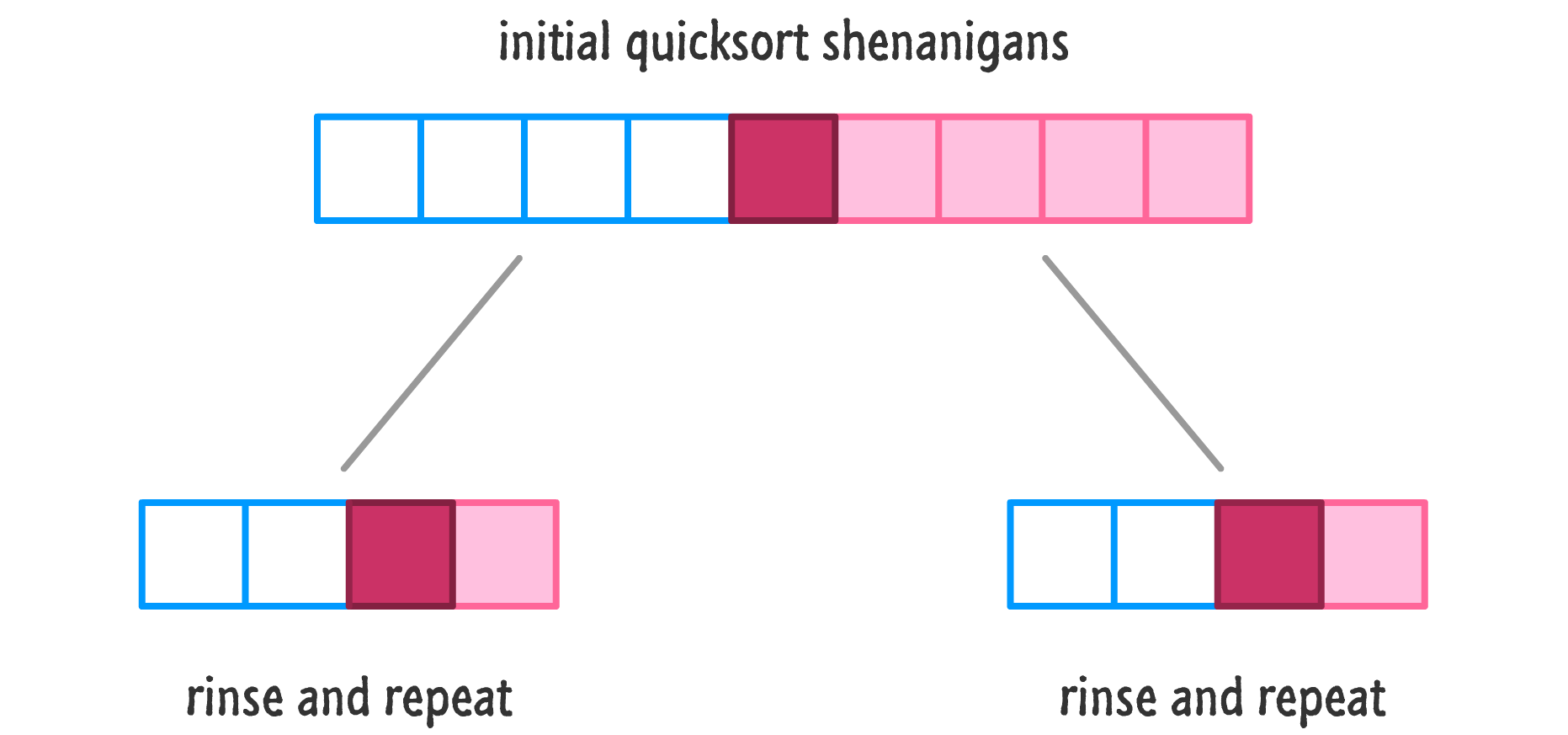You pick a pivot and then move values to either side of it to ensure smaller values are to the left and larger values are to the right. At this point, depending on how many items you are trying to sort, you'll have more regions of numbers that you need to divide and conquer.

Keep repeating all of this pivoting and sorting on each of the sections until you get to the point where you don't have enough values to pick a pivot and divide from: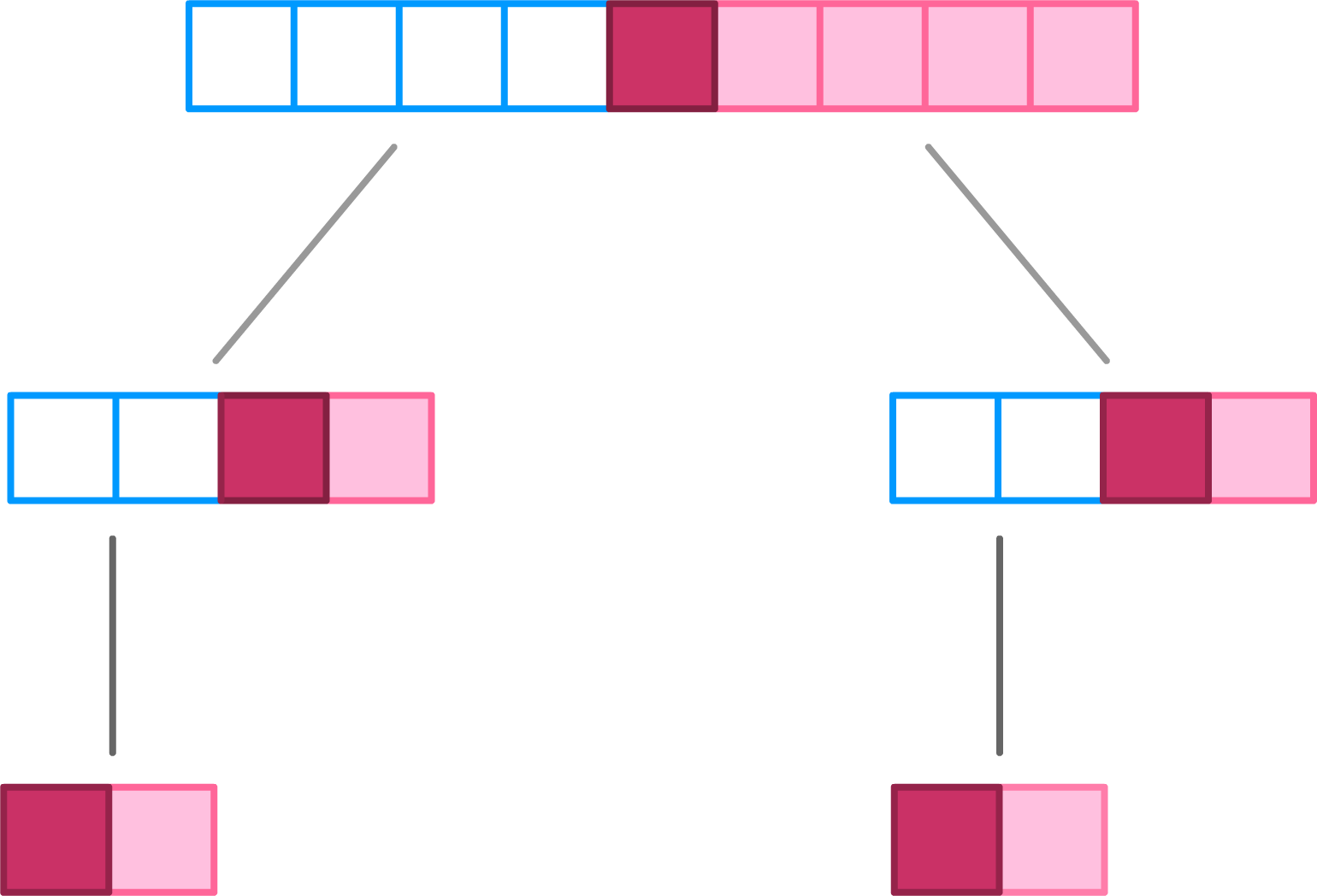Once you reach that point you an divide no further, guess what? You are done! Your initial collection of unordered data is now sorted from small to large. The reason for this seems cray cray, but take a few moments to think about what exactly happened at each stage. More specifically, think about how each pivot value, once everything got shuffled around, represented the final sorted location for that particular item.

Below is the progression as our list goes from being completely unsorted to being fully sorted with the the darker colors representing the pivot values and the bar above the numbers indicating the location that quicksort is currenly focusing its attention on: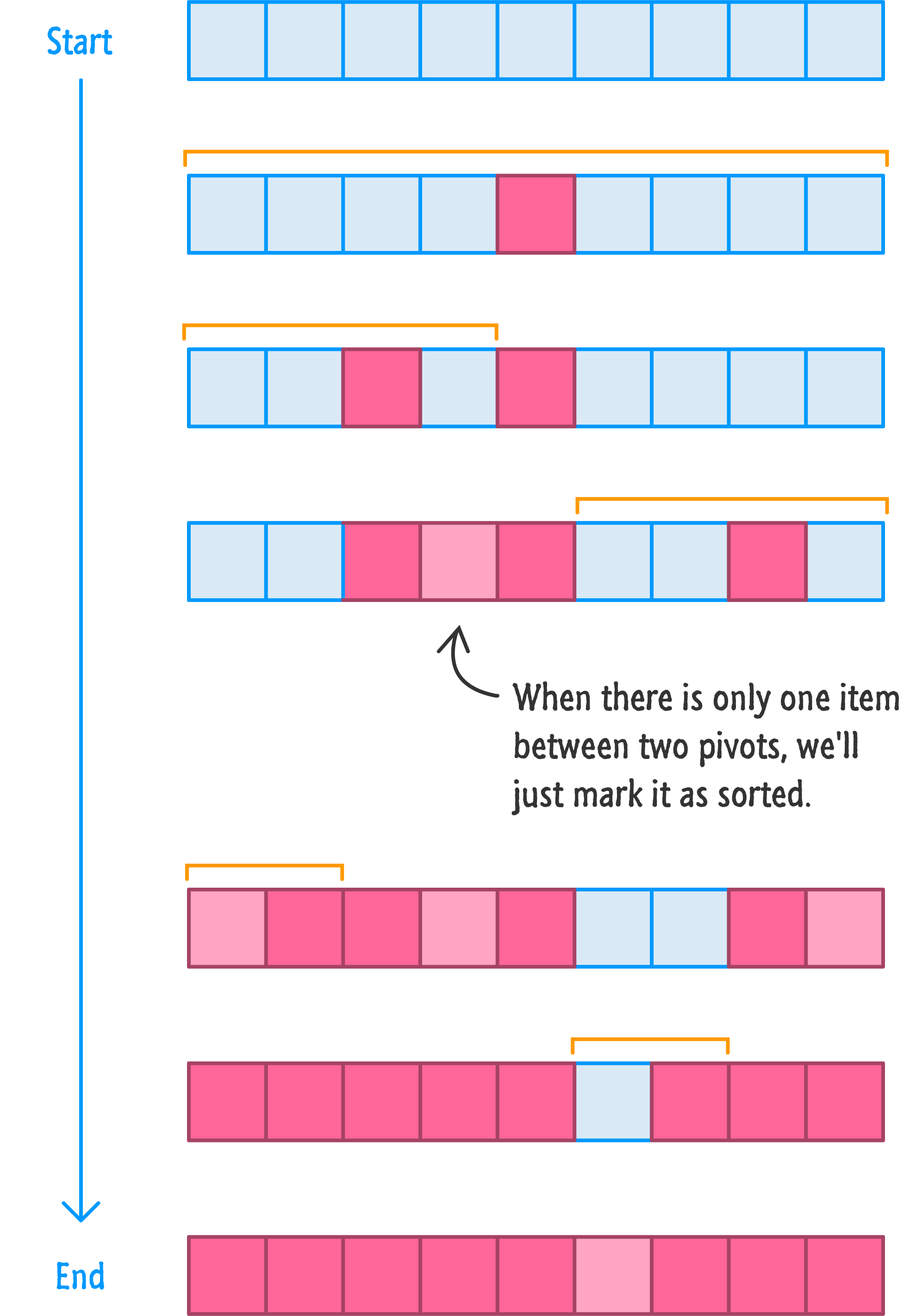By simply arranging items from "smaller than pivot" or "larger than pivot" and repeating this over and over again on each semi-ordered list until you only ended up with pivots, you end up slowly refining a bunch of data from semi-ordered to fully-ordered.

## A Slightly More Involved Look

The previous section was a high-level overview of how quicksort works. In my quest to get through it without throwing too many complexities at you, we glossed over a lot of subtle details that make a whole lot more sense now that you have a vague-ish idea of how quicksort works. In this section, let's fix that by continuing with the boxes from earlier. The major change is that, this time, let's give each box a value to better visualize what value each stores: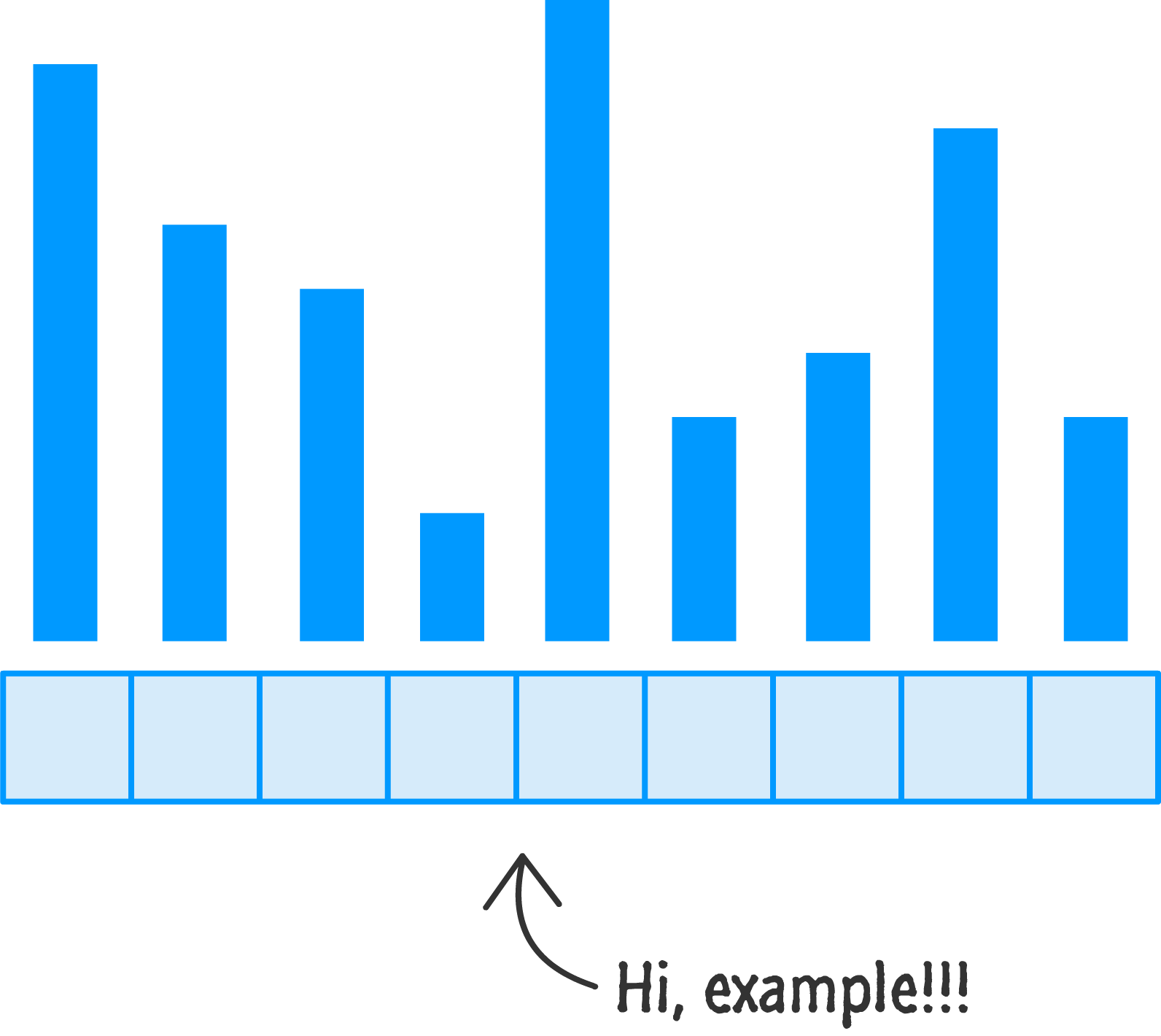The size (or magnitude) of the value is represented by the height of the bar. A taller bar indicates a larger value. A smaller bar indicates a smaller value. Let's take what we learned in the previous section and see how quicksort will help you sort this. Hopefully much of this will be a review.

As always, the first thing is for you (pretend you are quicksort!) to pick a pivot value, and we'll pick one in the middle: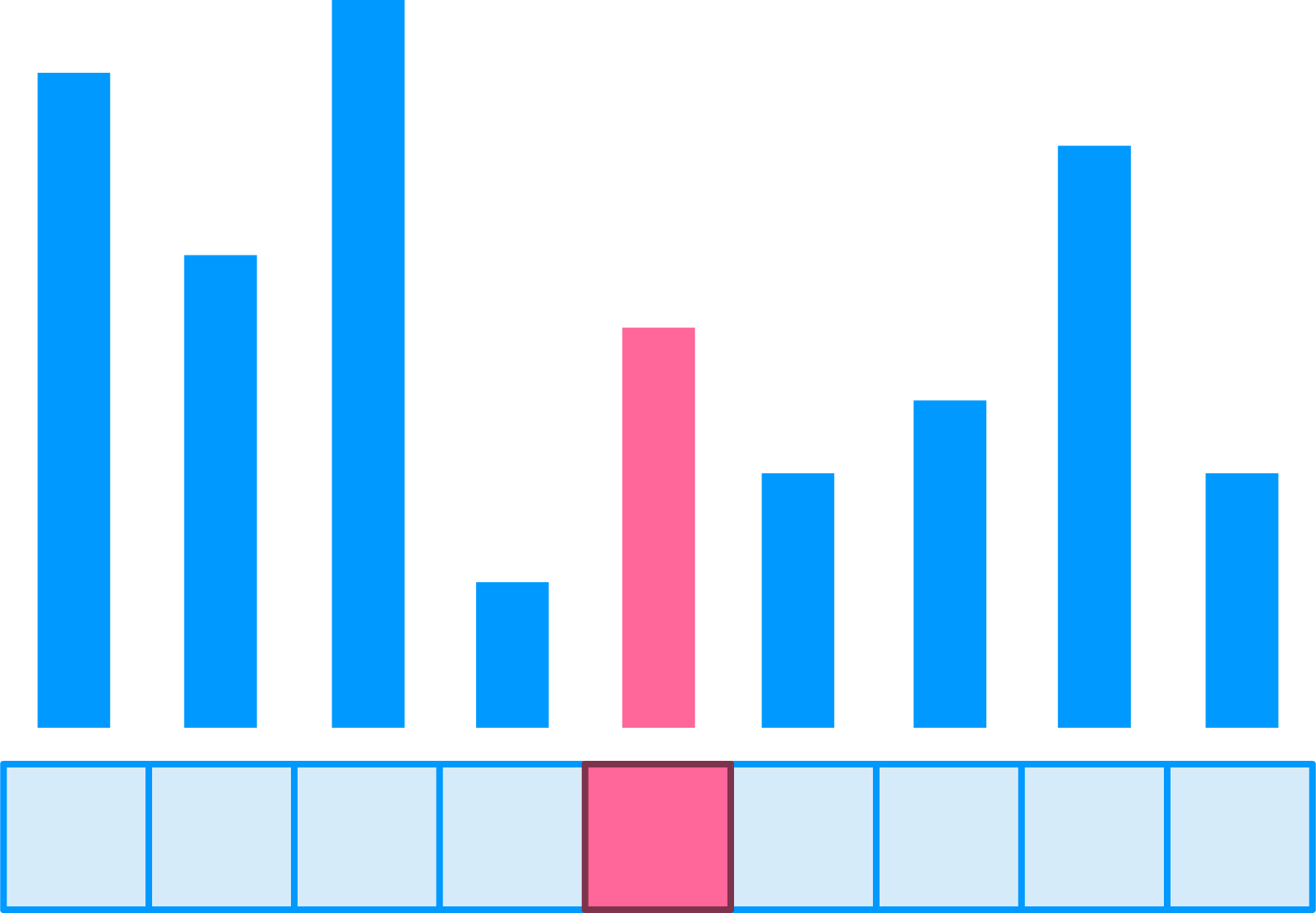Once the pivot has been picked, the next step is to move smaller items to the left and larger items to the right of the pivot: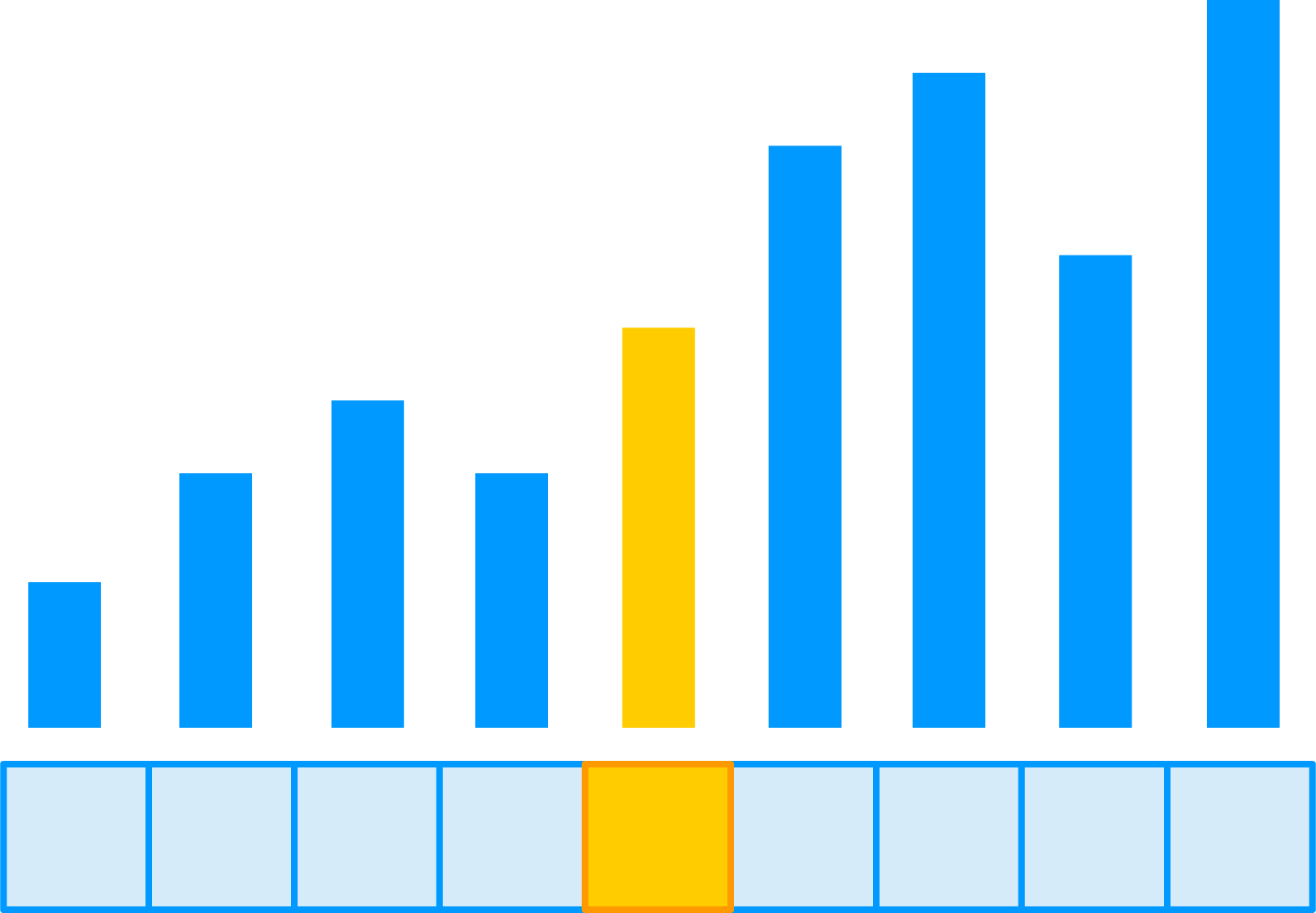At this point, your pivot value is considered to be sorted and in the proper location. After all, it is right in the middle of all the items that will appear before or after it. The next step is to sort the left half of the newly arranged items: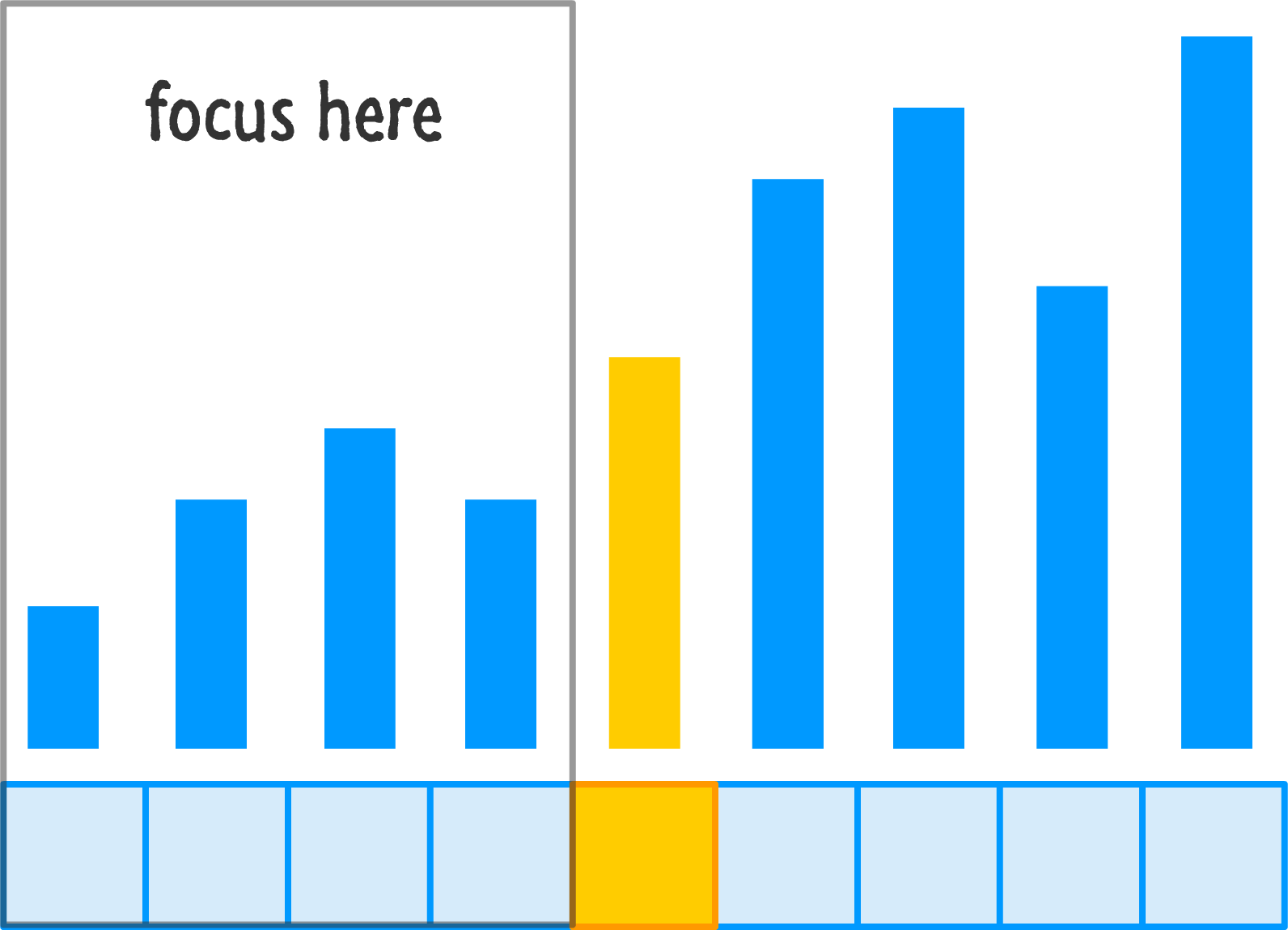This is done by recursively calling quicksort on just the left region. Everything that you saw before such as picking a pivot and throwing values around will happen again:The end result is that your left half is now semi-ordered and you have a smaller range of values left to arrange. Let's jump over to the right-half that we left alone after the first round of re-orderings and go mess with it: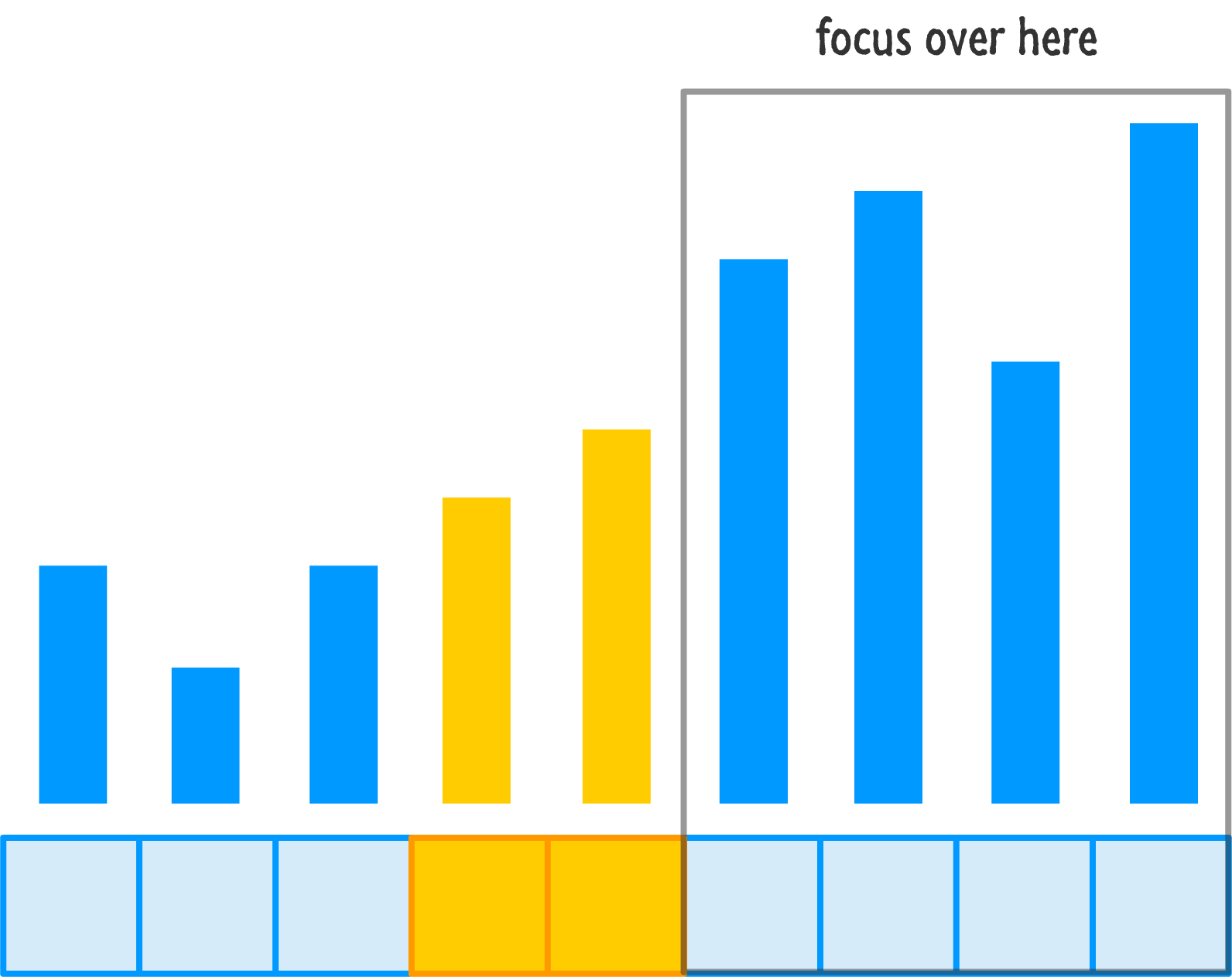Let's rinse and repeat our pivot and re-ordering steps on this side of our input:By now, you should be able to see the pattern more clearly. This means I can be lazy and skimp on the details by just showing you the remaining steps for getting our entire input properly ordered: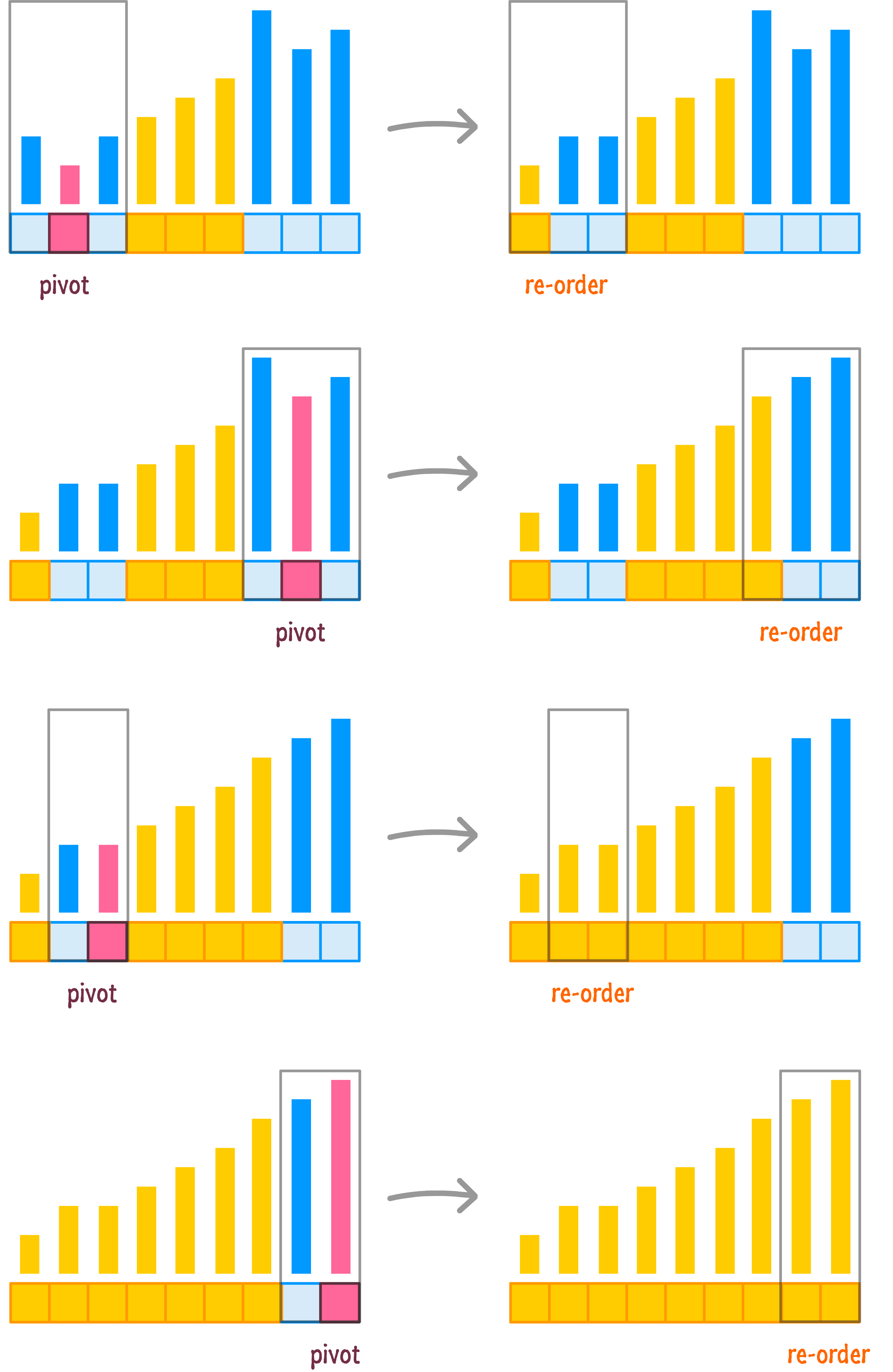As you can see, the end result of the various pivotings and re-orderings is that you get a fully ordered set of numbers in the end. Ok, now that you have seen all of this, you are in good shape to get even more detailed and start translating all of this into something your computers can actually do something with.

## Getting Technical

All of these words and diagrams are only helpful for people like you and me. Our computers have no idea what to do with all of this, so that means we need to convert everything we know into a form that computers understand. Before we go all out on that quest, let's meet everyone half-way by looking at some pseudocode (not quite real code, not quite real English) first.

The pseudocode for quicksort will look as follows: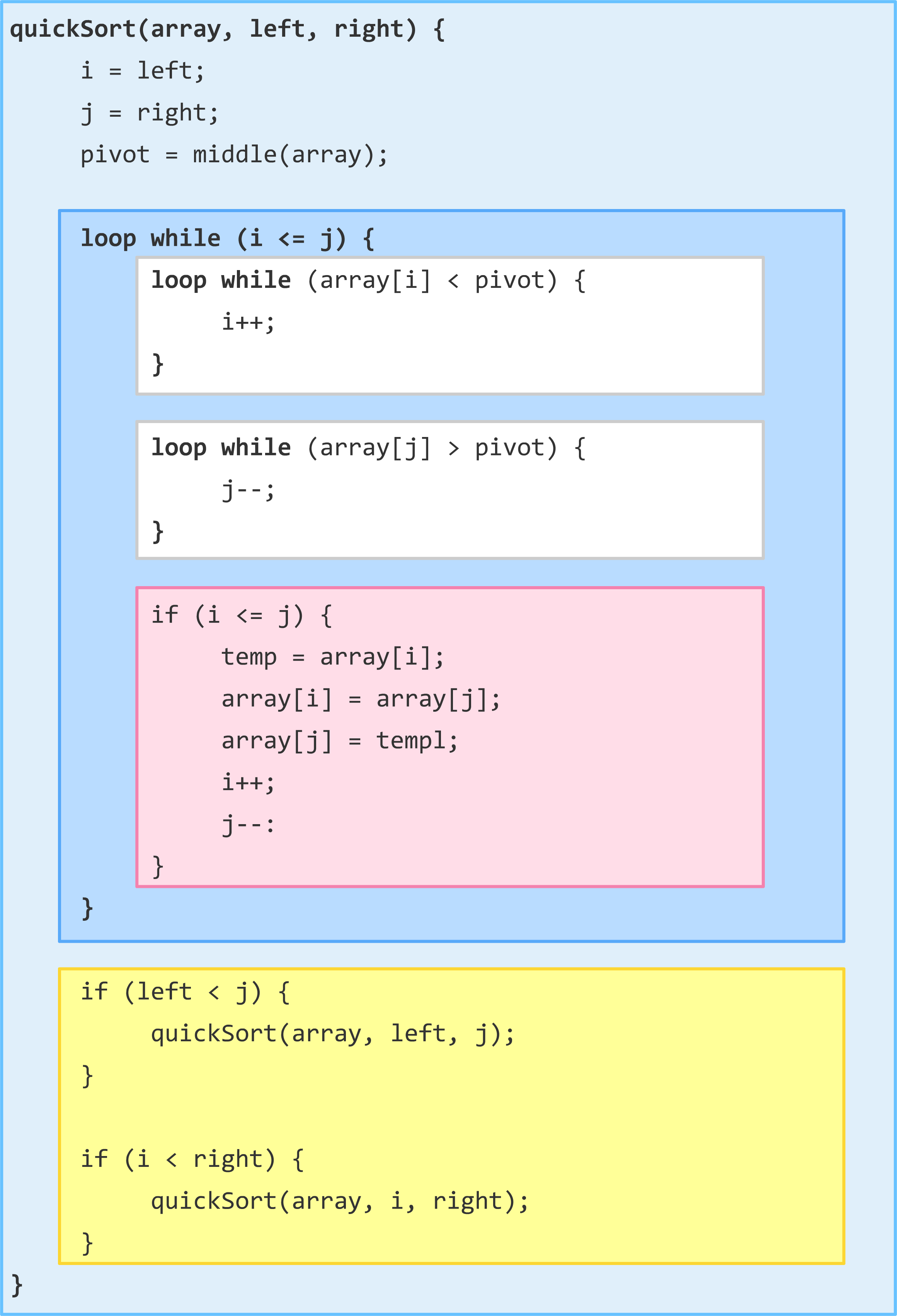Each of the colored regions represents an important step in what quicksort does. Not to give too much away, but here is a super-quick guide to what each chunk of code does: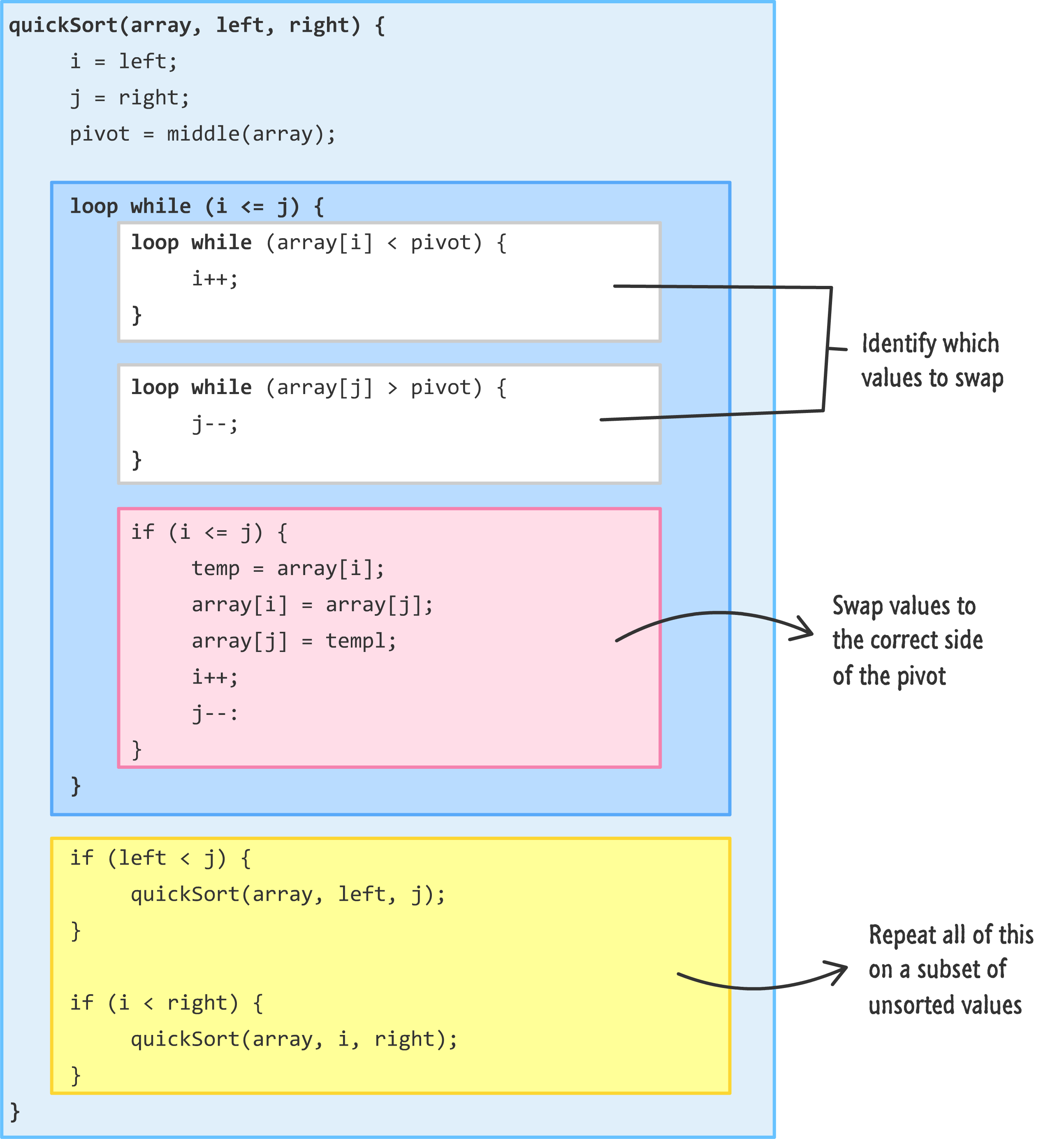Take a few moments to walk through how this code might work and how it might help you to sort an unsorted list of data. If this is confusing, don't worry. We'll go through it together starting...right...now!

### Step 1: The Pivot Value, Left Position, and Right Position

The first thing we will need to do is give our our quickSort function the things it needs to get started:

```quickSort(array, left, right) {
i = left;
j = right;
pivot = middle(array);

.
.
.
}```

The three things it needs are represented by the array, left, and right arguments. The array argument stands for the thing we want to sort, so here is our thing:This thing can be a series of values stored in any variety of data structures, but let's assume that what we want to sort is in the form of an array. The left and right arguments point to the start and end positions of what we are sorting. For the initial quicksort call, that is represented by the items at the first and last positions: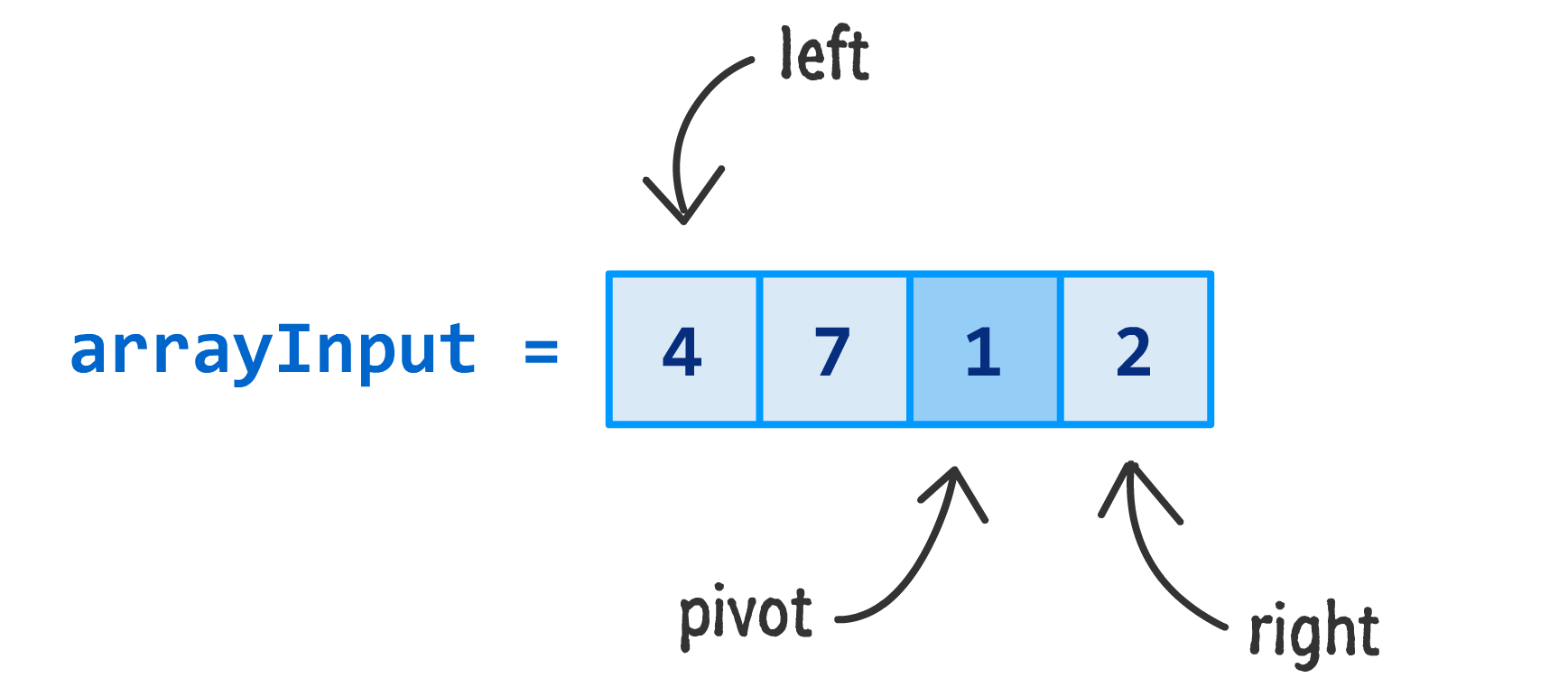Tying this all back to our code, the i variable points to the position of the first item in our our input passed in by the left argument. The j variable stores the position of the last item in our input as passed in by the right argument. The pivot variable stores the value of the middle point of our input:

If we had to explicitly call out these values, they would be as follows:

• i is 0
• j is 3
• pivot is 2

The main thing to note is that i and j point to index positions. They don't care what value is actually stored at that location. The pivot variable is different in that it does care and points to an actual value. This is an important detail to keep track of while we look at the rest of the code.

### Step 2: Determining What Values to Swap

Here is where things stand right now: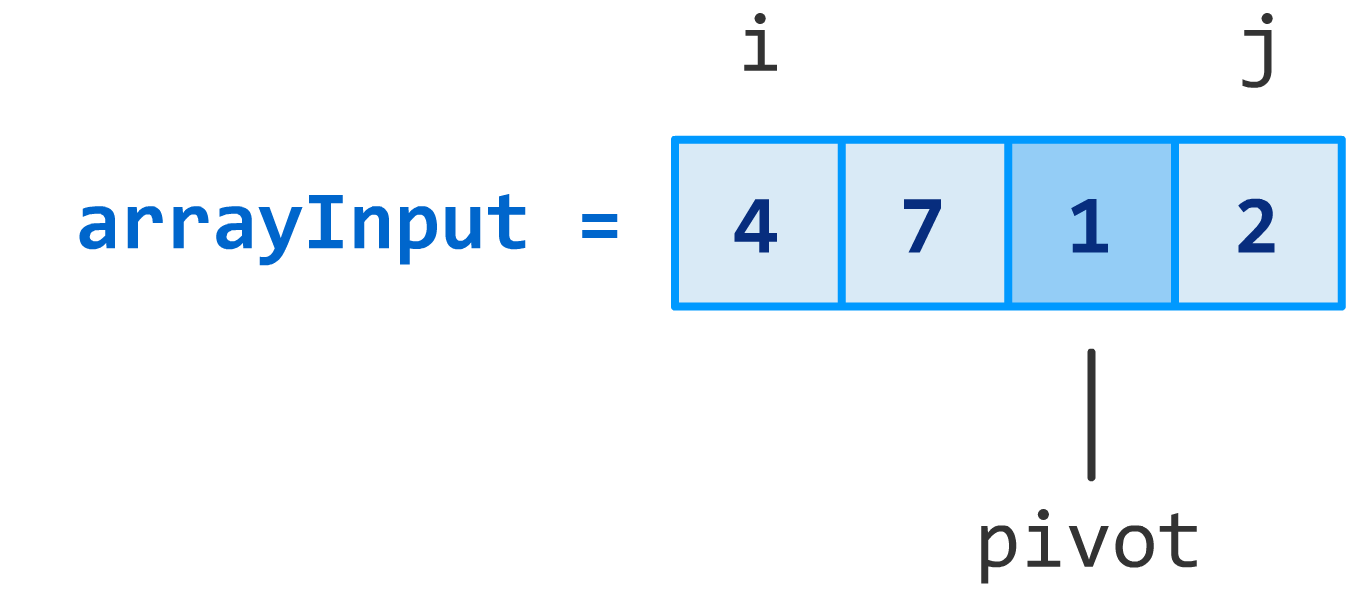The first thing to do once we've identified the pivot and the boundaries of the values in play is to figure out which elements need to be swapped around. As you may recall from the walkthroughs, a kerfuffle takes place where items on either side of the pivot are thrown around until everything:

1.  to the left of the pivot is smaller than the pivot value
2. to the right of the pivot is larger than the pivot value

Our i and j variables play an important role in this kerfuffle, and this kerfuffle is more formally known as the partition. Everything related to the partition is handled by the following code: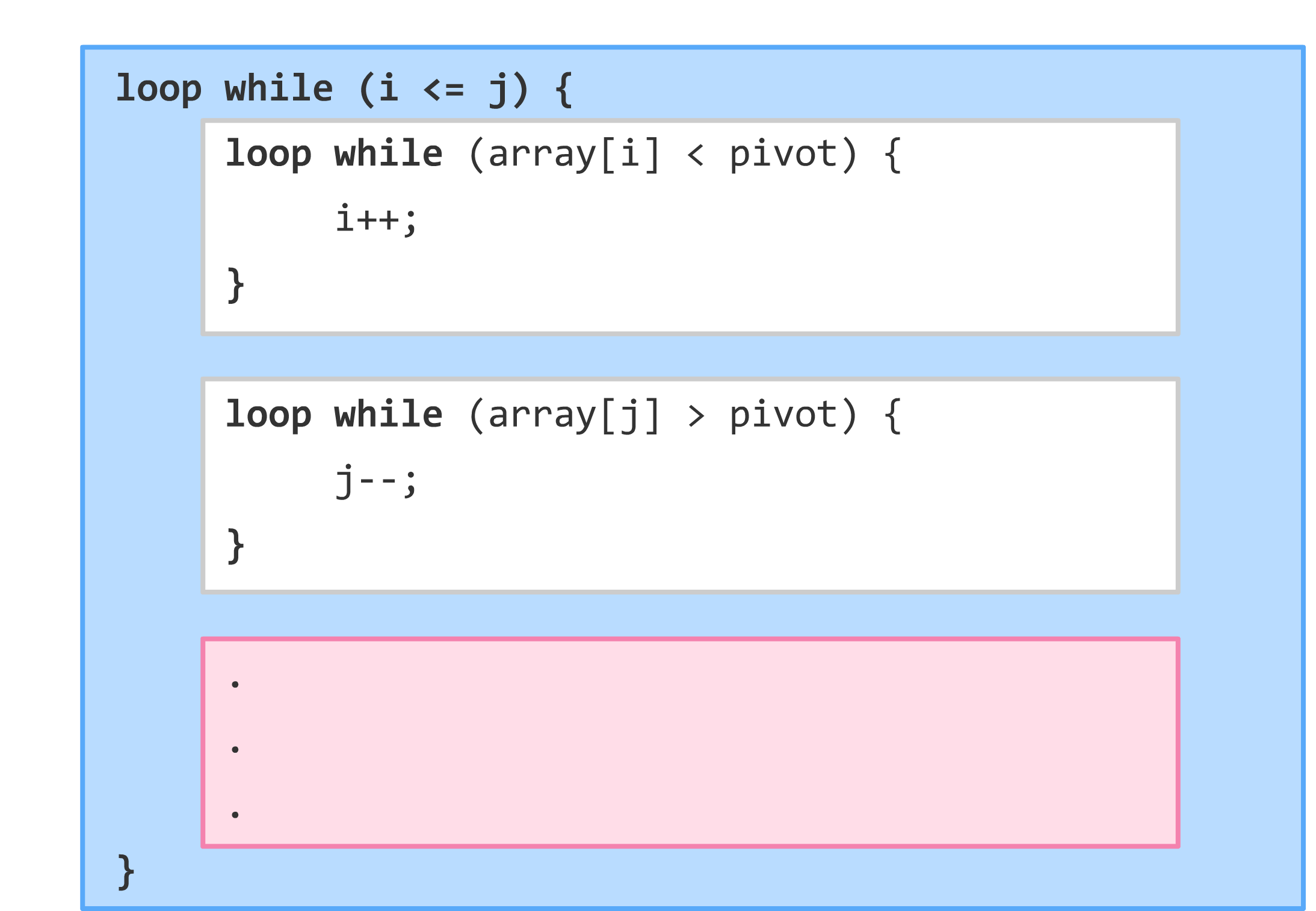You'll see three loops. The first outer loop ensures that i is less than j. This is important to ensure our loop doesn't run forever. Inside that outer loop, you have two inner loops (represented by the white boxes) that help determine which values need to be moved around:

```// left of pivot
loop while (array[i] < pivot) {
i++;
}

// right of pivot
loop while (array[j] > pivot) {
j--;
}```

Let's look at each loop individually.

#### The First Inner Loop

The first inner loop basically asks, "Is our current left array value less than our pivot value?" If the left array value is less than the pivot value, increase the value of i by 1. If the left array value is larger, drop everything and move on.

In our case, this is what everything looks like right now:Let's take stock of what the values for everything is. The value of i is 0, array[i] is 4, and pivot is 1. Is the value of array[i] less than pivot? In other words, is 4 less than 1? The answer is clearly a nope. We'll need to deal with this by (spoiler alert!) throwing the current value at position i to the other side of our pivot to ensure nothing larger than the pivot appears to the left of it, but we'll get there shortly.

#### The Second Inner Loop

At this point, we exit the first inner loop and run into our second inner loop:

```// right of pivot
loop while (array[j] > pivot) {
j--;
}```

This loop is similar to the earlier one, but it runs only if the value of our array at the position indicated by j is greater than the value of pivot: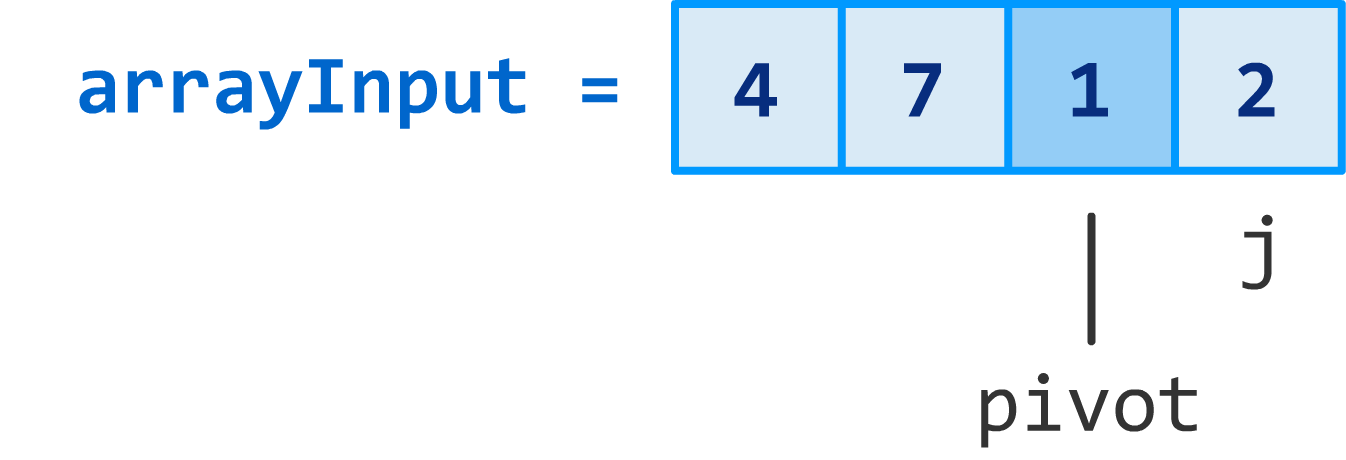The value of j is the index position of the last item in our array, and that is 3. The value of array[j] is clearly 2, and the pivot value is still 1. The answer to this loop's condition is...yes! Our array[j] is greater than pivot, so we reduce the value of j by 1:

```// right of pivot
loop while (array[j] > pivot) {
j--;
}```

Our loop runs one more time, and this time our j's index position is one less than what it was before:At this point, you can see that our loop's condition no longer holds true. The value of array[j] is equal to the pivot. It isn't greater than, so this loop also ends.

Both our inner loops have run to completion, and the values of the interesting pieces at this point are:

• i is 0
• j is 2
• array[i] is 4
• array[j] is 1
• pivot is 1

Visually, this looks as follows: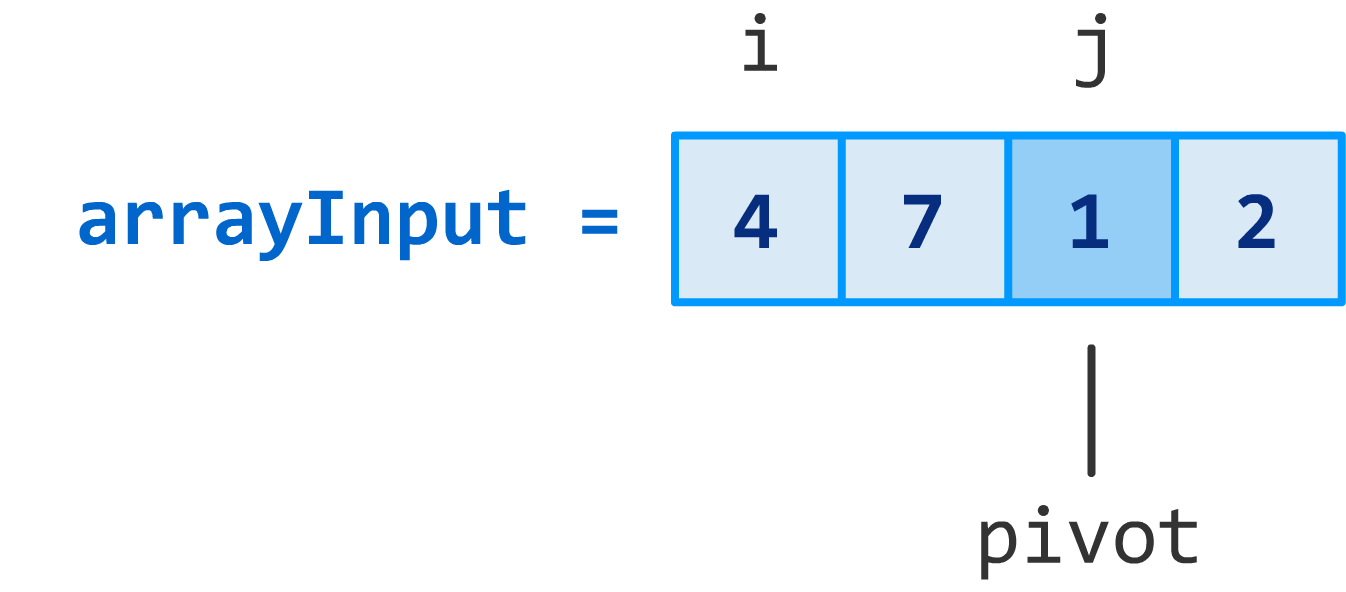It might seem like all of this resulted in nothing much happening, but as you will see shortly, that isn't entirely accurate.

### Step 3: Actually Swapping the Values

The thing that remains at this point is to swap the values we identified that need swapping to ensure our pivot is in the middle. That is handled by the last chunk of (non-grayed out) code: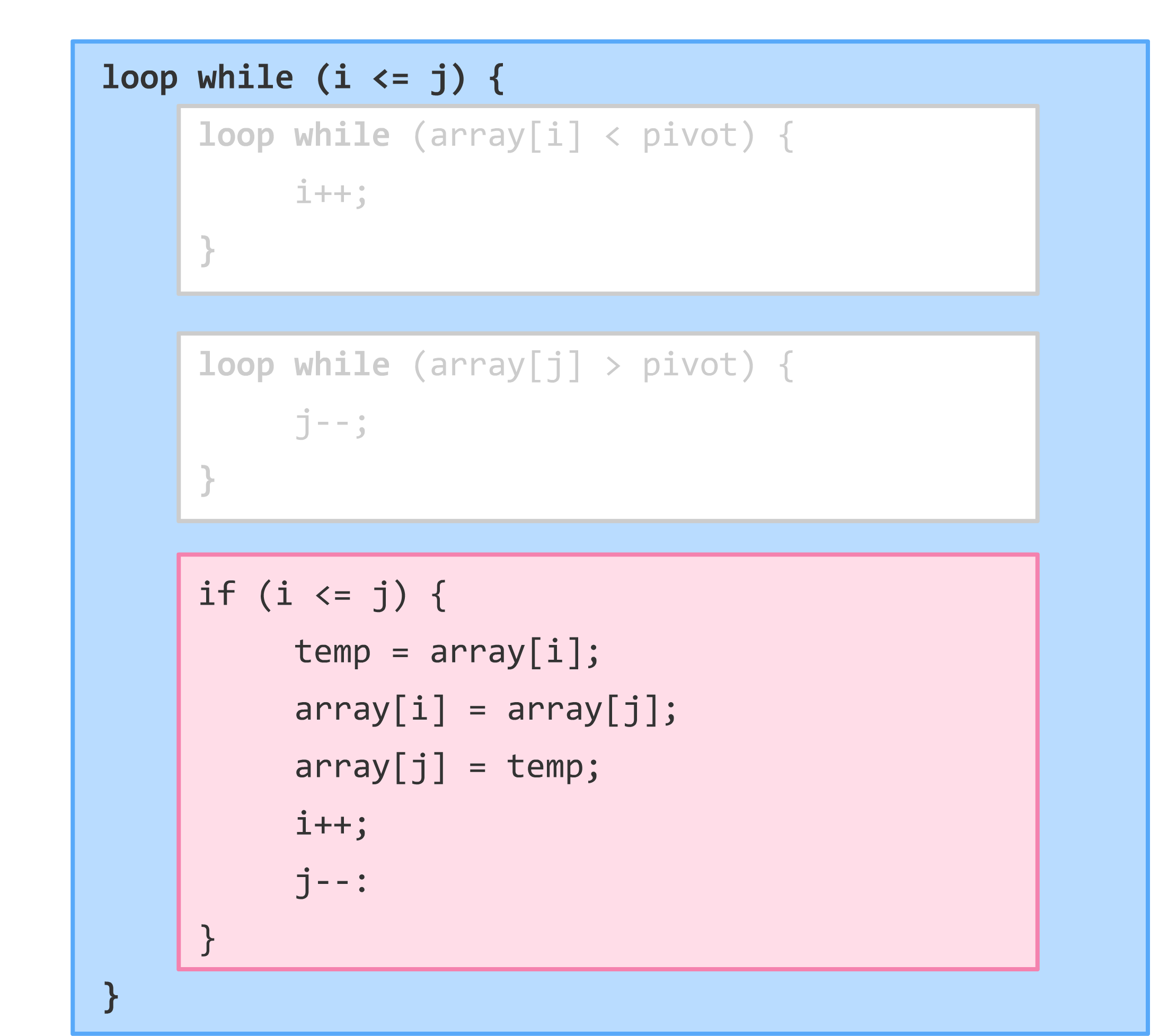Swapping values is pretty easy. The temp variable stores the current value of the item referenced by i. The swap happens in the next set of lines where the value of array[i] and array[j] is set to each other's value (with temp playing a helping hand):

```if (i <= j) {
temp = array[i];
array[i] = array[j];
array[j] = temp;
i++;
j--:
}```

After the swap, something very important happens. The value of i is increased by 1, and the value of j is decreased by 1. Here is a visualization of what things look like:Notice that our pivot value was involved with the swap, so it is no longer in the middle like it once was. That's totally OK, for your pivot value will shift as items are inserted or removed around it in more complex examples...such as this one, as it turns out. Lastly, shifting i and j results in both of them now pointing to the second item.

### Step 4: Continue Swapping Numbers Around

Swapping values to either side of the pivot isn't a one-time thing as you saw earlier. This goes on until all items to the left of the pivot are smaller and all items to the right of the pivot are larger. This is why the code for making all of this work lives inside a loop:The value of and i and j are both 1, so the condition this outermost loop relies on is still valid. All of the steps you've seen so far repeat one more time. I won't walk through everything in detail again, but I will run through it quickly one more time so that you can see how the values change.

Right now, these are the variables and their values:

• i is 1
• j is 1
• array[i] is 7
• array[j] is 7
• pivot is 1

If we go through the code, the conditions set by our first inner loop will fail:

```// left of pivot
loop while (array[i] < pivot) {
i++;
}```

The value of array is 7, and it is definitely not less than our pivot's value which is 1. So let's skip this. Next up is the second inner loop:

```// right of pivot
loop while (array[j] > pivot) {
j--;
}```

The value of array[j] is also 7, but this condition checks if it is greater than the value of pivot. As it turns out, 7 is greater than 1, so we decrease the value of j. The values of everything at this point is:

• i is 1
• j is 0
• array[i] is 7
• array[j] is 1
• pivot is 1

The value of j and array[j] have changed as a result of this loop. They are now 0 and 1 respectively. At this point, our loop's condition will fail:

```// right of pivot
loop while (array[j] > pivot) {
j--;
}```

The j variable points to the same location as pivot, so the values of pivot and array[j] are equal. This loop is done, so we move on to this code that checks if i is less than or equal to j:The value of i is not less than j, so the condition fails. We are now back to the outer loop, and the outer loop sets forth the same condition to see if i is less than or equal to j. Clearly nothing changed in the few milliseconds to change anything, so this condition also fails.

We are done with the swapping operation with all elements to the left of our pivot being less than the pivot itself AND all elements to the right of our pivot being greater than the pivot itself: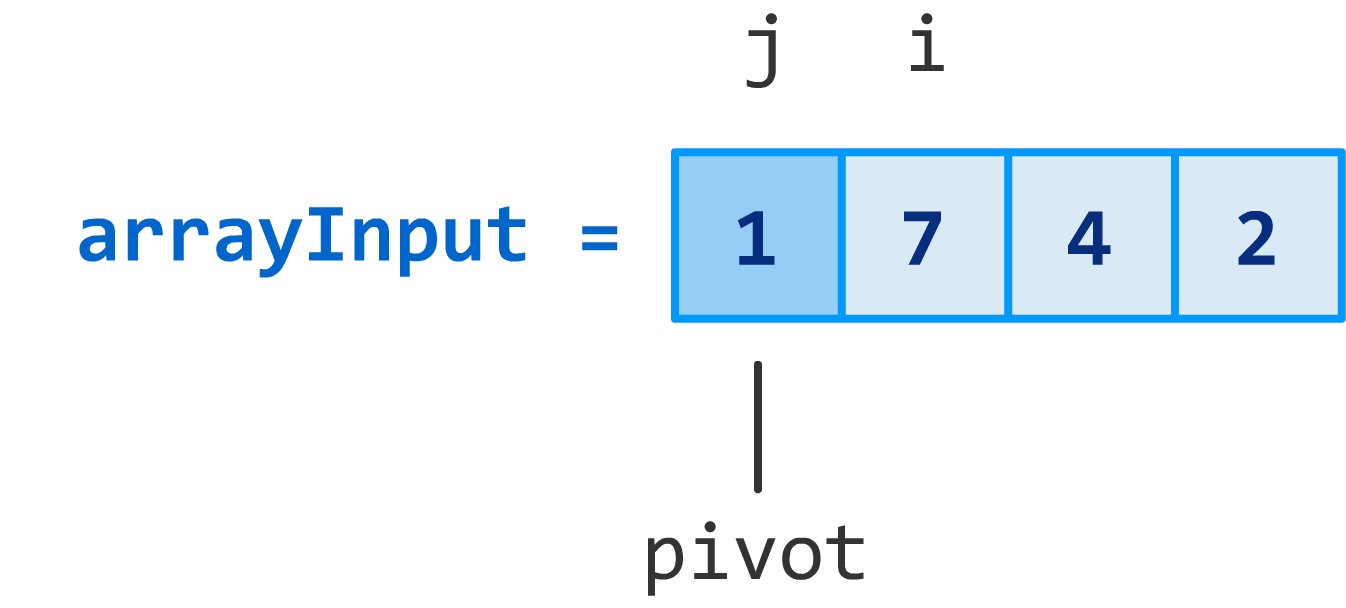We have finally escaped the clutches of all these loops...for now.

### Step 5: Recursively Calling Quicksort Again

We are almost done with this call to quicksort. Remember, we need to sort the remaining partially sorted items by doing the whole pivot picking and swapping work (aka the partition) all over again:This means that, while we are done with this call to quicksort, we will end up having to make more calls to sort the rest of the partially sorted data. All of that is determined by the following chunk of code:The first condition asks if the value of left from waaay back when is less than j. Since left is 0 and j is 0, the first condition fails.

The second condition asks if the value of i (which is 1) is less than right (which is 3). Hot diggity! That condition is true, so we call quicksort all over again:

```if (i < right) {
quickSort(array, i, right);
}```

The major difference is that this time we are scoping things down to only the values constrained by i and right...which happens to the be same as the partially sorted region from earlier: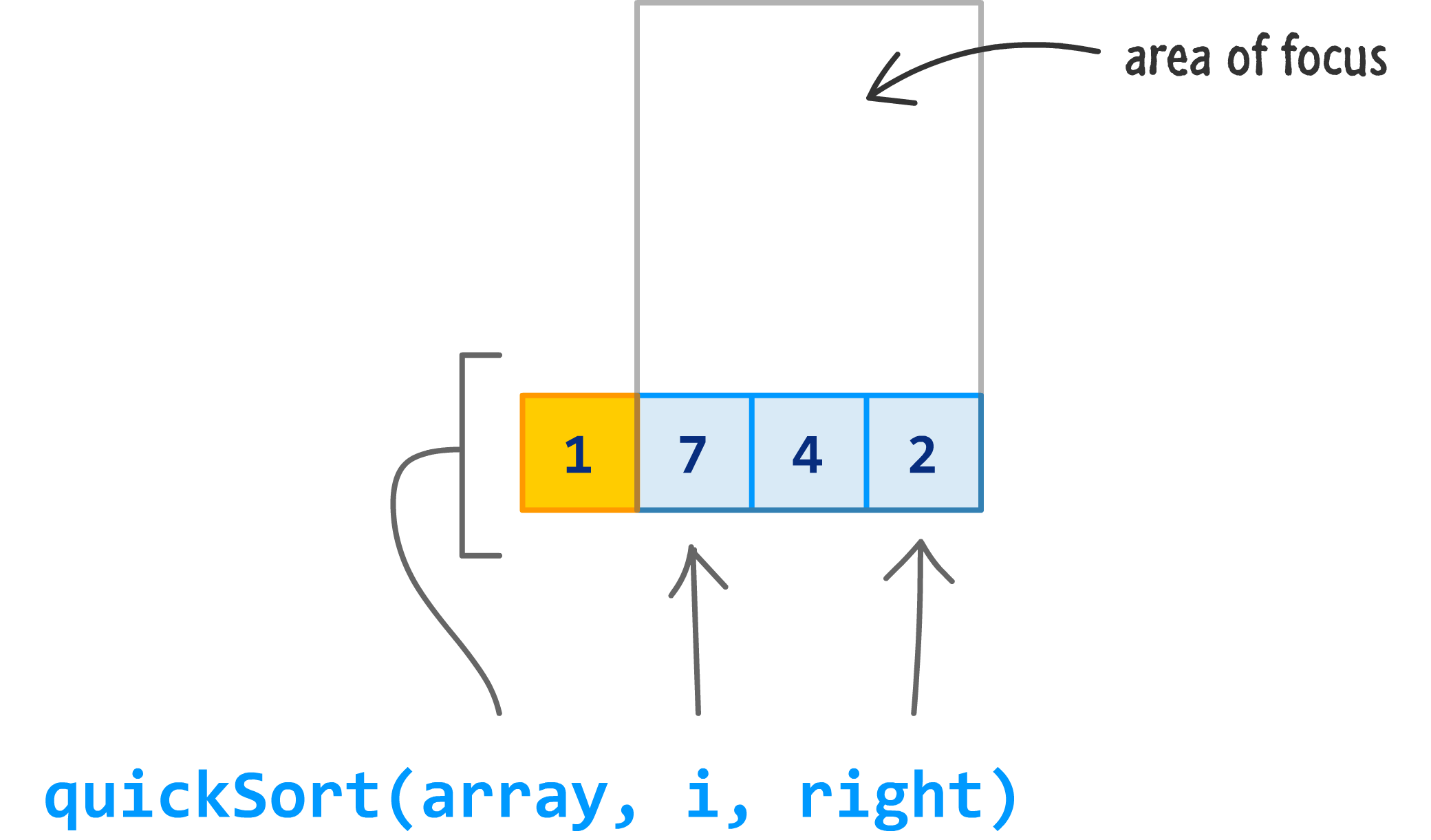When quicksort gets called again, the values for pivot, left, right, i, j, and everything else will be reset to take into account this new range of data. From there on out, your code goes back to Step 1 and runs through everything over again (and maybe even a few times more!) until you have no more partially sorted values left.

The end result is a fully sorted input of data: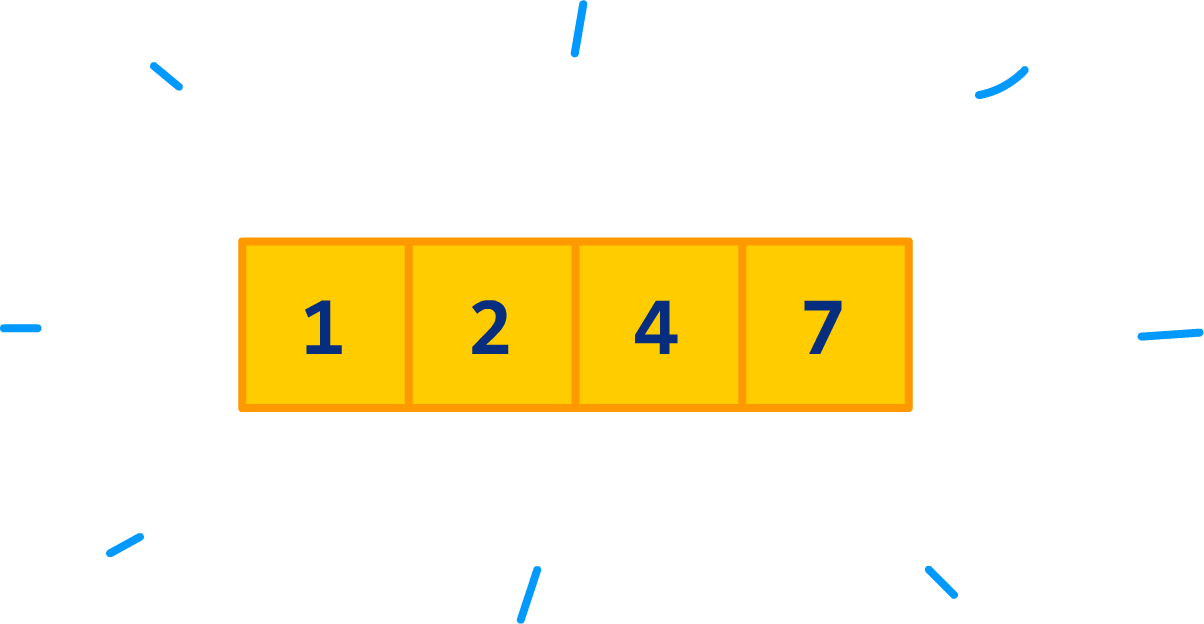Everything you've seen so far works for one item, four items, or a million items. The five steps quicksort uses remain unchanged.

## Some Actual Code

Phew. If you made it this far, you learned how quicksort works by looking at many MANY pages of text, diagrams, and even some pseudocode. All that remains is to look at an actual implementation of quicksort that you can use:

```function quickSortHelper(arrayInput, left, right) {
let i = left;
let j = right;
let pivotPoint = arrayInput[Math.round((left + right) * .5)];

// Loop
while (i <= j) {

while (arrayInput[i] < pivotPoint) {
i++;
}

while (arrayInput[j] > pivotPoint) {
j--;
}

if (i <= j) {
let tempStore = arrayInput[i];

arrayInput[i] = arrayInput[j];
i++;

arrayInput[j] = tempStore;
j--;
}

}

// Swap
if (left < j) {
quickSortHelper(arrayInput, left, j);
}

if (i < right) {
quickSortHelper(arrayInput, i, right);
}

return arrayInput;
}

function quickSort(input) {
return quickSortHelper(input, 0, input.length - 1);
}```

The code you see here is largely identical to the pseudocode you saw earlier. The biggest change is that I created a quickSortHelper function to deal with specifying the array, left, and right values. This makes the call to the quickSort function very clean. You just specify the array.

Here is an example of how to use this code:

```let myData = [24, 10, 17, 9, 5, 9, 1, 23, 300];
quickSort(myData);

If everything is setup correctly (and why wouldn't it?!!), you'll see a dialog displaying a sorted list of numbers.

## Conclusion

Well, you have reached the end of this dive into one of the fastest sort algorithms. Will all of this knowledge help you out in real (non-academic) life? I highly doubt it. Almost all popular programming languages have their own built-in sort mechanism that you can use. Many are already based on quicksort (or a highly optimized and specialized version of it), so the performance gains you will see by using your own version of quicksort compared to using a built-in sort approach will be zero.

In that case, why did we spend so much time on this? Besides the obvious reasons of entertaining people with your newfound knowledge, one of the reasons is that the built-in sort mechanisms will fail you at some point. You may find yourself needing to sort a more complex set of data that goes beyond what the built-in sorts support. At times like that, you may have to implement your own sort algorithm. What you implement may be based on quicksort or it may be something completely unrelated. Speaking of unrelated, below is a table comparing various popular sorting algorithms and their various performance and memory characteristics:

Name Best Average Worst Memory
Quicksort n log n n log n n2 log n (average)
Mergesort n log n n log n n log n n (worst case)
Heapsort n log n n log n n log n 1
Timsort n n log n n log n n
Bubble sort n n2 n2 1
Selection sort n2 n2 n2 1
Insertion sort n n2 n2 1

You can find a more detailed comparison of these sorting algorithms as well as many others over on the Wikipedia section fully dedicated to this.

And with that, you are free to go and use your newfound knowledge to sort all sorts of things really REALLY quickly.

Got a question or just want to chat? Comment below or drop by our forums (they are actually the same thing!) where a bunch of the friendliest people you'll ever run into will be happy to help you out!